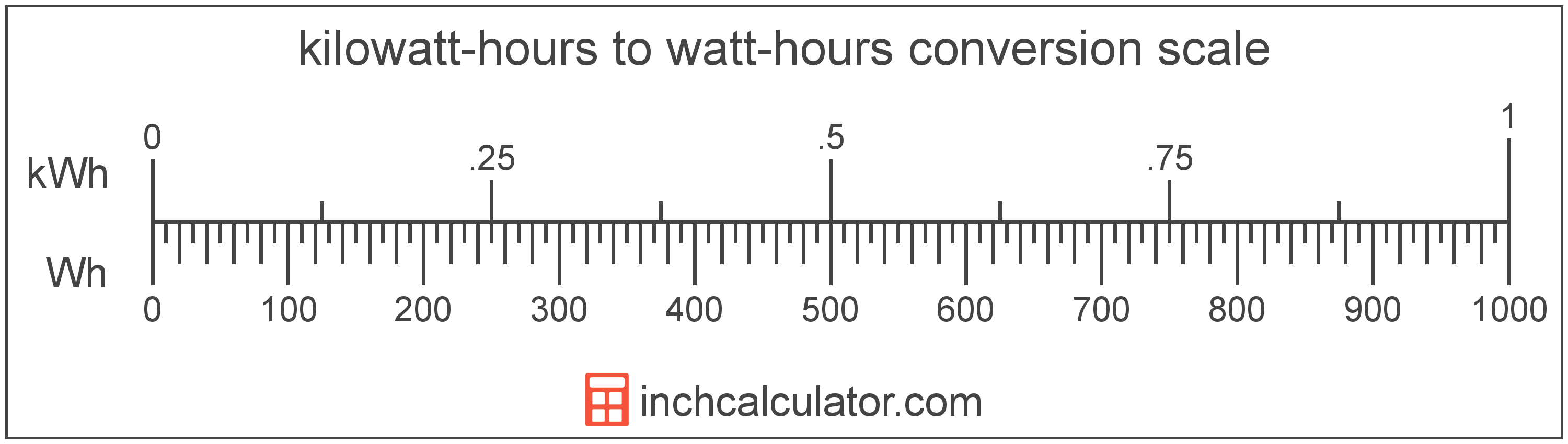# Kilowatt-Hours to Watt-Hours Converter

Enter the energy in kilowatt-hours below to get the value converted to watt-hours.

Results in Watt-Hours:1 kWh = 1,000 Wh

Do you want to convert watt-hours to kilowatt-hours?

## How to Convert Kilowatt-Hours to Watt-Hours

To convert a measurement in kilowatt-hours to a measurement in watt-hours, multiply the energy by the following conversion ratio: 1,000 watt-hours/kilowatt-hour.

Since one kilowatt-hour is equal to 1,000 watt-hours, you can use this simple formula to convert:

watt-hours = kilowatt-hours × 1,000

The energy in watt-hours is equal to the energy in kilowatt-hours multiplied by 1,000.

For example, here's how to convert 5 kilowatt-hours to watt-hours using the formula above.
watt-hours = (5 kWh × 1,000) = 5,000 Wh### How Many Watt-Hours Are in a Kilowatt-Hour?

There are 1,000 watt-hours in a kilowatt-hour, which is why we use this value in the formula above.

1 kWh = 1,000 Wh

## What Is a Kilowatt-Hour?

A kilowatt-hour is a measure of electrical energy equal to one kilowatt, or 1,000 watts, of power over a one hour period. Kilowatt-hours are a measure of electrical work performed over a period of time, and are often used as a way of measuring energy usage by electric companies.

Kilowatt-hours are usually abbreviated as kWh, although the formally adopted expression is kW·h. The abbreviation kW h is also sometimes used. For example, 1 kilowatt-hour can be written as 1 kWh, 1 kW·h, or 1 kW h.

In formal expressions, the centered dot (·) or space is used to separate units used to indicate multiplication in an expression and to avoid conflicting prefixes being misinterpreted as a unit symbol.

## What Is a Watt-Hour?

The watt-hour is a measure of electrical energy equal to one watt of power over a one hour period.

Watt-hours are usually abbreviated as Wh, although the formally adopted expression is W·h. The abbreviation W h is also sometimes used. For example, 1 watt-hour can be written as 1 Wh, 1 W·h, or 1 W h.

## Kilowatt-Hour to Watt-Hour Conversion Table

Table showing various kilowatt-hour measurements converted to watt-hours.
Kilowatt-hours Watt-hours
0.001 kWh 1 Wh
0.002 kWh 2 Wh
0.003 kWh 3 Wh
0.004 kWh 4 Wh
0.005 kWh 5 Wh
0.006 kWh 6 Wh
0.007 kWh 7 Wh
0.008 kWh 8 Wh
0.009 kWh 9 Wh
0.01 kWh 10 Wh
0.02 kWh 20 Wh
0.03 kWh 30 Wh
0.04 kWh 40 Wh
0.05 kWh 50 Wh
0.06 kWh 60 Wh
0.07 kWh 70 Wh
0.08 kWh 80 Wh
0.09 kWh 90 Wh
0.1 kWh 100 Wh
0.2 kWh 200 Wh
0.3 kWh 300 Wh
0.4 kWh 400 Wh
0.5 kWh 500 Wh
0.6 kWh 600 Wh
0.7 kWh 700 Wh
0.8 kWh 800 Wh
0.9 kWh 900 Wh
1 kWh 1,000 Wh

## References

1. Bureau International des Poids et Mesures, The International System of Units (SI), 9th edition, 2019, https://www.bipm.org/documents/20126/41483022/SI-Brochure-9-EN.pdf